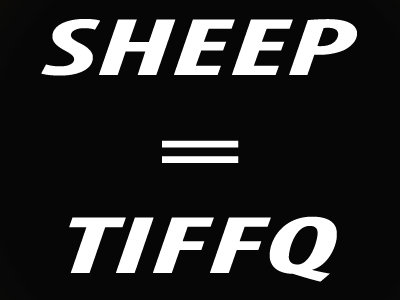Learn how to crack letter for letter codes.

# VR - Codes Using Letters for Letters

Codes Using Letters for Letters are basically what they say they are - codes in which letters are used to represent other letters. In the past, codes were used to pass on secret messages so that anybody unfamiliar with the code could not read the meaning of the message. That’s exactly how Letters for Letters codes work in Verbal Reasoning – though they should be much easier to decipher!

## How Are Letters for Letters Codes Used In The Exam?

There are three different formats codes take in the Verbal Reasoning Exam. Codes in which letters are represented by other letters are slightly different to the other two, which we will look at in later exam illustrations.

In Letters for Letters codes, candidates are shown a five-letter word, along with its representation in a code, which looks like a random combination of letters. They are then shown another five-letter word and asked to find the way this word is shown in the same code.

Occasionally, the questions may be reversed and, rather than being asked to find how a word would be formed as a code, candidates are instead shown a coded word and asked to decipher it.

Let’s look at some examples of codes using letters for letters:

Example Question One

If the code for CREAM is DSFBN then what is the code for BANKS?

So how can the code be worked out? The transposition of letters is what it's all about; crack the code of how many places are being moved along the alphabet and you'll probably be able to see the answer.

In this type of question, relate the first letter of the first word, ‘cream’, to the first letter of the second word, ‘dsfbn’.

Next, look at the second letter of each. As you will find, this is a simple code where each of the code letters is one along in the alphabet from the original. In other words, C becomes D, R becomes S and so on. This is pretty straightforward - there won't be anything quite so easy in the real test!

Now we can work out the answer – B must become C, A becomes B, N becomes O, K becomes L and S becomes T. The code for BANKS is therefore CBOLT. Remember, the 'code' does not have to make sense as a word!

Example Question Two

If the code for BOOK is AQNM then what is the code for TYPE?

Let’s start by dealing with it letter by letter and applying the same rule for the first pair of words to the second: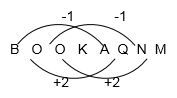As you can see, the pattern is not simple like it was in example one. However, it does follow a regular pattern, albeit an alternating one. If we apply a similar rule for each letter of TYPE then we get the following: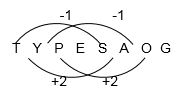Again, be aware that the alphabet in these questions is a loop – go one letter back from A and you get Z! The answer to the question is SAOG.

Don't forget that, as it is a 'code' that we are looking for, there is no need to be finding a legitimate 'word' as the answer. If there was a question working the other way round, and you had a code but needed to find a 'word', then you must check that it is a recognisable word!

Example Question Three

If the code for TIME is SGJA then what is LYFH the code for?

Let’s apply the same process as we did in the previous example but this time we need to do things in reverse to find the answer.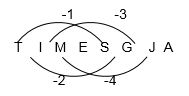The first code letter - S - is one back from T. The second is two back, then three, then four. In order to find the answer, we need to go from the new code back to the word it must represent. Therefore, we have to go in reverse. Instead of reaching the code we need to reach the word that created it.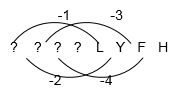To find the first letter, we must add one back on to the code letter ‘L’. The second letter is found by adding two back on to ‘Y’ (remembering that the alphabet is on a loop).

The answer will look like this: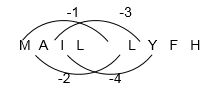Therefore, the answer is M A I L.

## Sample Tests

So, now that you have learnt how to crack letter for letter codes in Verbal Reasoning, it’s time to put your child to the test.

There are four quizzes on the Education Quizzes site devoted specifically to this type of question. Try them with your child. If necessary, show them the tips we have learned in this lesson to guide them.

You’ll find the quizzes in our Eleven Plus Verbal Reasoning section or, alternatively, you can follow these links:

Related Words 1

Related Words 2

Related Words 3

Related Words 4

The more familiar your child becomes with deciphering codes, the less intimidating they will find these question when they come to face them. Practise is key so, happy code-cracking!